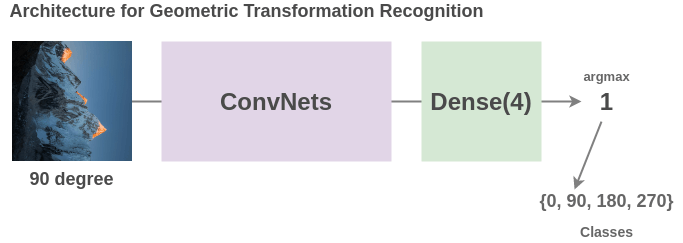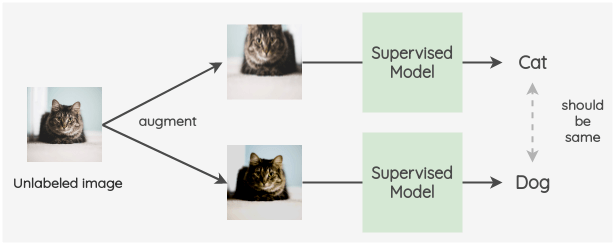What is the relation between semi-supervised and self-supervised visual representation learning?

What's the differences between semi-supervised learning and self-supervised visual representation learning, and how they are connected?

Both semi-supervised and self-supervised methods are similar in the sense that the goal is to learn with fewer labels per class. The way both formulate this is quite different:

1. Self-Supervised Learning:

This line of work aims to learn image representations without requiring human-annotated labels and then use those learned representations on some downstream tasks. For example, you could take millions of unlabeled images, randomly rotate them by either 0, 90, 180 or 270 degrees and then train a model to predict the rotation angle. Once the model is trained, you can use transfer learning to fine-tune this model on a downstream task like cat/dog classification just like how you finetune ImageNet pretrained models. You can view an overview of the methods and also look at contrastive learning methods that are currently giving state-of-the-art results such as SimCLR and PIRL.1. Semi-supervised Learning

Different from self-supervised learning, semi-supervised learning aims to use both labeled and unlabeled data at the same time to improve the performance of a supervised model. An example of this is FixMatch paper where you train your model on labeled images. Then, for your unlabeled images, you apply augmentations to create two images for each unlabeled image. Now, we want to ensure that the model predicts the same label for both the augmentations of the unlabeled images. This can be incorporated into the loss as a cross-entropy loss.Semi-supervised learning

Semi-supervised learning is the collection of machine learning techniques where there are two datasets: a labelled one and an unlabelled one.

There are two main problems that can be solved using semi-supervised learning:

• transductive learning (i.e. label the given unlabelled data) and
• inductive learning (generalization) (i.e. find a function that maps inputs to outputs, like classification).

Self-supervised learning

Self-supervised learning (SSL) is a machine learning approach where the supervisory signal is automatically generated. More precisely, SSL can either refer to

• learn data representations (i.e. learn to represent the data) by solving a so-called pretext (or auxiliary) task, in a self-supervised fashion, i.e. you automatically generate the supervised signal from the unlabelled

• automatically label an unlabelled dataset by exploiting data coming from different sensors (this is the usual definition of SLL in the context or robotics)

What is the relationship between the two?

SSL (for data representation) can be considered a semi-supervised learning approach, if you fine-tune the learned data representations with a labeled dataset to solve supervised learning problem (i.e. the so-called downstream task), which you will probably do otherwise the data representations are pretty useless. Read this answer for other details.

The previous answer has given a good insight into the difference between two areas. I would like to give more examples.

Semi-Supervised Learning work with improving the data set by adding up new examples. There are iterative systems where we train a model on a given dataset and improve the model further after deploying it on the real world by adding interactions of the real world and their outcomes to further train the system.

Self-Supervised Learning is becoming a very hot topic these days. It has the ability to understand the underline properties of a given dataset with some kind of a supervisory signal (Not exactly a label). self-Attention introduced in Transformers is a modern day popular self-supervised learning. Also, check this tweet from Yann Lecun tweet